Question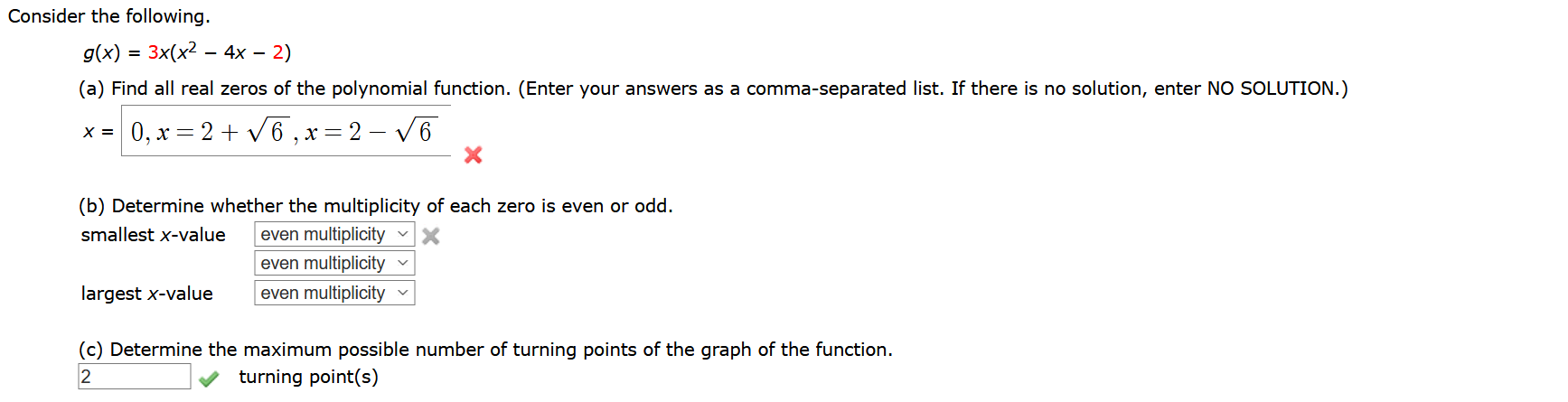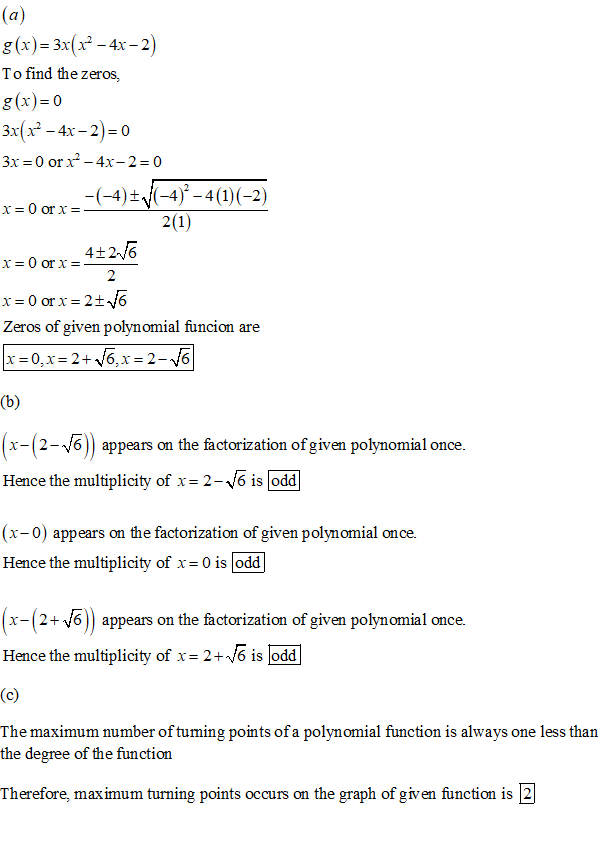#### Earn Coins

Coins can be redeemed for fabulous gifts.

Similar Homework Help Questions
• ### Given f (x) = x4 + 5x3 – 24x2, find all real zeros off and determine...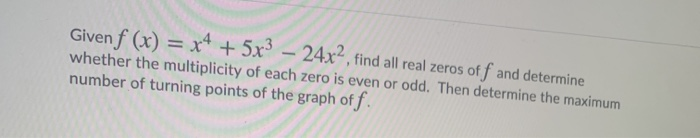Given f (x) = x4 + 5x3 – 24x2, find all real zeros off and determine whether the multiplicity of each zero is even or odd. Then determine the maximum number of turning points of the graph off.

• ### Given the graph of a polynomial function, determine the minimum possible degree, the zeros and if...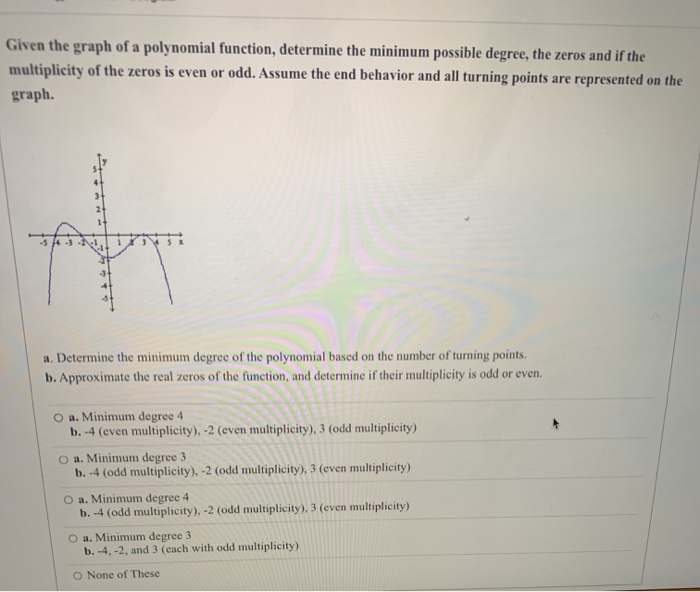Given the graph of a polynomial function, determine the minimum possible degree, the zeros and if the multiplicity of the zeros is even or odd. Assume the end behavior and all turning points are represented on the graph. གནད་ a. Determine the minimum degree of the polynomial based on the number of turning points. b. Approximate the real zeros of the function, and determine if their multiplicity is odd or even O a. Minimum degree 4 b. -4 (even multiplicity),...

• ### Analyze the polynomial function f(x)=x2 + x2 - 20x. Complete parts (a) through (c). (a) Find...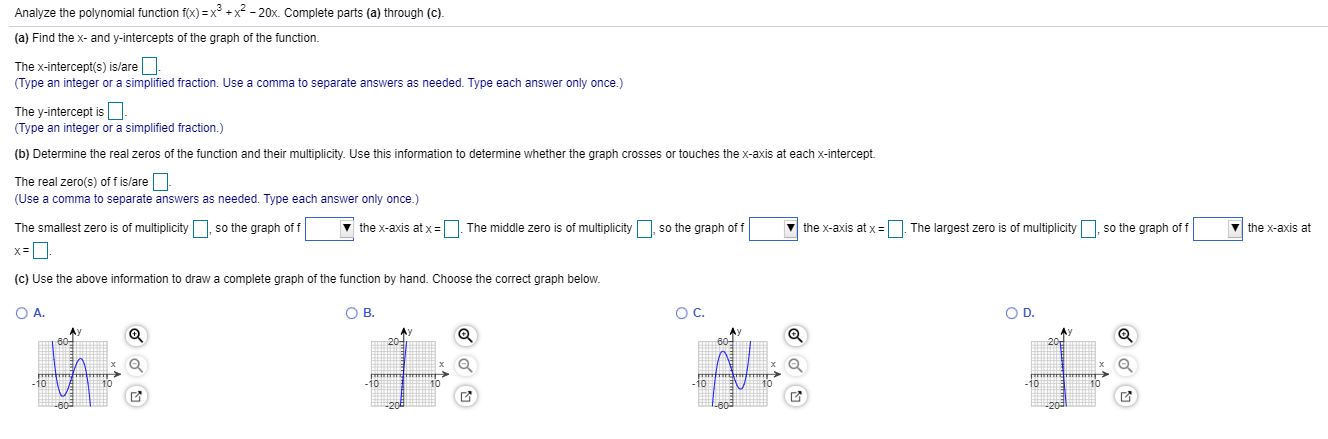Analyze the polynomial function f(x)=x2 + x2 - 20x. Complete parts (a) through (c). (a) Find the x- and y-intercepts of the graph of the function. The x-intercept(s) is/are - (Type an integer or a simplified fraction. Use a comma to separate answers as needed. Type each answer only once.) The y-intercept is - (Type an integer or a simplified fraction.) (b) Determine the real zeros of the function and their multiplicity. Use this information to determine whether the graph...

• ### Consider the following polynomial function. f(x) = – 8x10 + 2 (a) Determine the maximum number...

Consider the following polynomial function. f(x) = – 8x10 + 2 (a) Determine the maximum number of turning points of the graph of the function. (b) Determine the maximum number of real zeros of the function. Consider the following polynomial function. f(x) = 6x5 + 3x4 + 5 (a) Determine the maximum number of turning points of the graph of the function. turning point(s) (b) Determine the maximum number of real zeros of the function. Consider the following polynomial function....

• ### Consider the following polynomial function. f(x) = – 8x10 + 2 (a) Determine the maximum number...

Consider the following polynomial function. f(x) = – 8x10 + 2 (a) Determine the maximum number of turning points of the graph of the function. (b) Determine the maximum number of real zeros of the function. Consider the following polynomial function. f(x) = 6x5 + 3x4 + 5 (a) Determine the maximum number of turning points of the graph of the function. turning point(s) (b) Determine the maximum number of real zeros of the function. Consider the following polynomial function....

• ### Find all the real zeros of the polynomial function and state their multiplicities.36

Find all the real zeros of the polynomial function and state their multiplicities.f(x)=2/7x^5-3/7x^4+1/4x^3? is a zero of multiplicity ?

• ### Find the zeros of the polynomial function and state the multiplicity of each zero. (Enter your...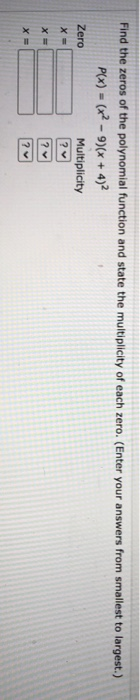Find the zeros of the polynomial function and state the multiplicity of each zero. (Enter your answers from smallest to largest.) P(x) = (x2 - 9)(x + 4)2 Zero Multiplicity ? X = X = ? х ? Evaluate the determinant by expanding by cofactors. 3-4 6 3-4 0 0 5 MOO

• ### Find the zeros for the given polynomial function and give the multiplicity for each zero. State...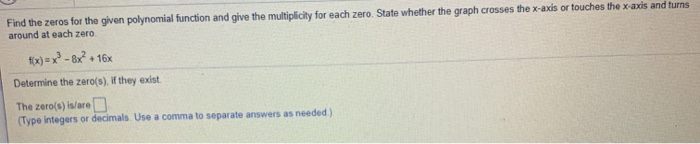Find the zeros for the given polynomial function and give the multiplicity for each zero. State whether the graph crosses the x-axis or touches the x-axis and turns around at each zero fix)=x2-8x? + + 16x Determine the zero(s). If they exist. The zero(s) is/are D (Type integers or decimals. Use a comma to separate answers as needed) Find the zeros for the given polynomial function and give the multiplicity for each zero. State whether the graph crosses the x-axis...

• ### Determine if the graph can represent a polynomial function. If so, assume the end behavior and...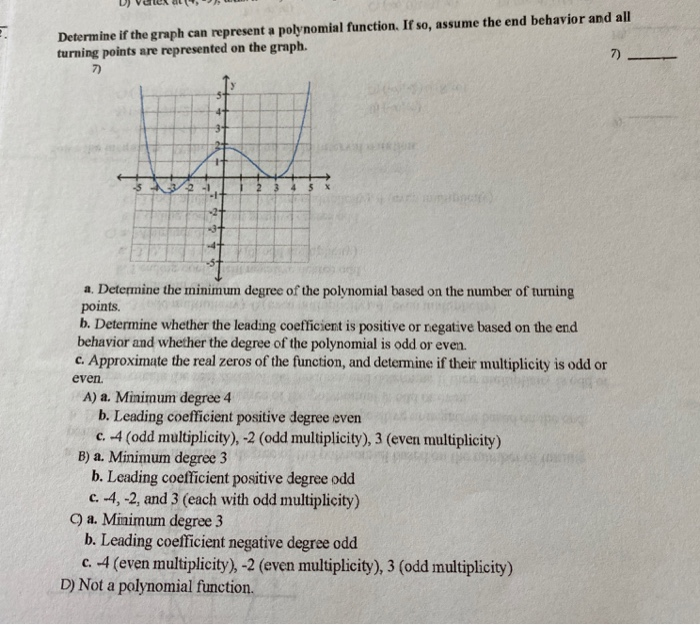Determine if the graph can represent a polynomial function. If so, assume the end behavior and all turning points are represented on the graph 7) 7) 2 a. Determine the minimum degree of the polynomial based on the number of turning points. b. Determine whether the leading coefficient is positive or negative based on the end behavior and whether the degree of the polynomial is odd or even c. Approximate the real zeros of the function, and determine if their...

• ### Find the zeros of the polynomial function and state the multiplicity of each. f(x) = 4x²-x²...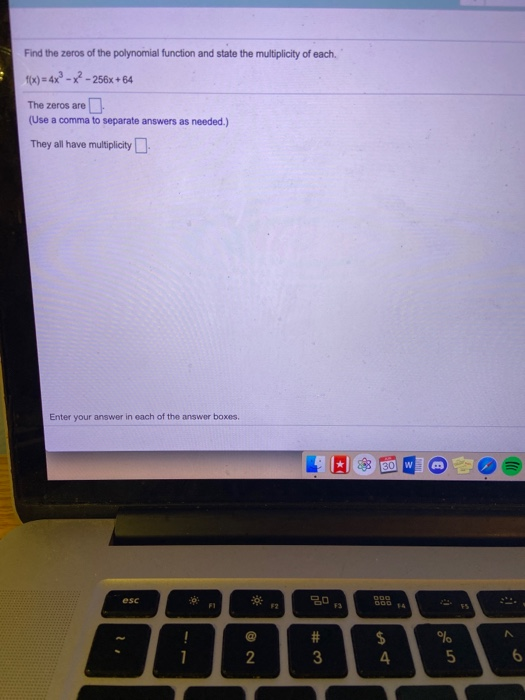Find the zeros of the polynomial function and state the multiplicity of each. f(x) = 4x²-x² - 256x+64 The zeros are (Use a comma to separate answers as needed.) They all have multiplicity Enter your answer in each of the answer boxes 30 W esc 30 ... 52 73 DOD # 3 \$ 4 % 5 6 N